• VBA示例之 从字符串提取数字，供初学者参考，大牛勿进~~~~~~~
• Private Sub CommandButton1_Click() s = Range("J2") Set regex = CreateObject("vbscript.regexp") With regex .Global = True .Pattern = "\d+" ' .Pattern = "[\u4e00-\u9fa5]+" For Eac...
Private Sub CommandButton1_Click()  s = Range("J2")  Set regex = CreateObject("vbscript.regexp")  With regex      .Global = True      .Pattern = "\d+"      ' .Pattern = "[\u4e00-\u9fa5]+"      For Each te In .Execute(s)         txt = txt & te      Next  End With  Range("l2") = txt
txt="" End Sub  
展开全文• 如下要提取单元格里得数字，怎么才能实现？很简单，一个自定义函数马上搞定。 代码如下 在VBE编辑窗口插入一个模块，记得是要在模块上写函数代码。 选择插入菜单，选择过程的函数，命名函数名（根据自己的喜好定义...
看到这个有博友将对这种简单的方法都要设置付币才能下载查看，我觉得太low了，知识只有分享才能走得更远。如下要提取单元格里得数字，怎么才能实现？很简单，一个自定义函数马上搞定。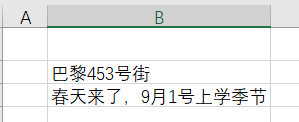代码如下 在VBE编辑窗口插入一个模块，记得是要在模块上写函数代码。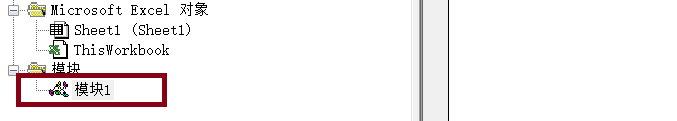选择插入菜单，选择过程的函数，命名函数名（根据自己的喜好定义函数名称，建议使用见文生义的命名方式）。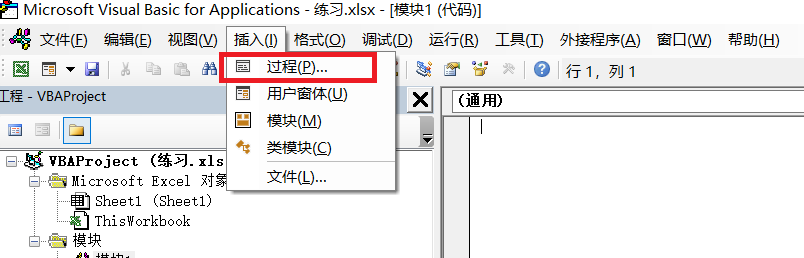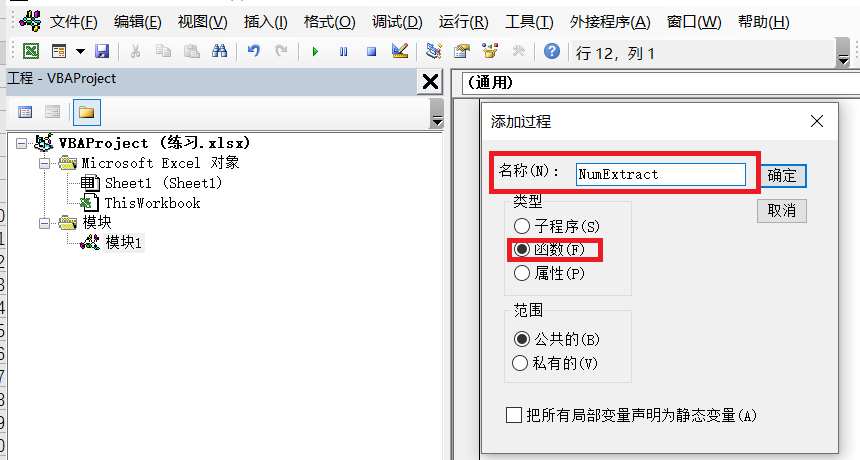实现的代码如下：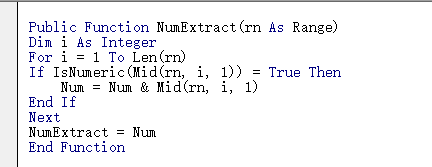这样函数就完成了，任意单元格里输入函数=NumExtract(要提取的单元格)，就可以将数字提取出来，当然还有其它方法实现，这里只给大家提供其中一个解决的方法。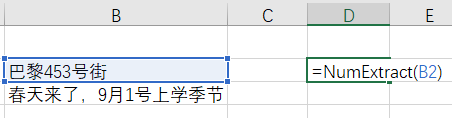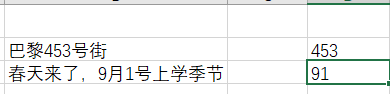是不是很简单？
展开全文经验分享
• 上一篇讲了用 python 提取字符串中的数字，这次用VBA来事项。总体思路和 python 是一样的，代码如下： Option Explicit Sub GetNumbers() Dim from_string As String, convert_numbers As String Dim i, j, k, ...
上一篇讲了用 python 提取字符串中的数字，这次用VBA来实现。总体思路和 python 是一样的，代码如下：
Option Explicit

Sub GetNumbers()

Dim from_string As String, convert_numbers As String
Dim i, j, k, m, first_number_location
Dim i1 As String
Dim check_start(9) As String, check_end(10) As String
Dim split_string() As String, get_numbers() As String

' 给 from_string 赋值
from_string = Cells(1, 1)
from_string = CStr(from_string)

' 先求出 check_start 和 check_end
' 用于后续检验 from_string 中每个字符是否是数字
For i = 0 To 9
i1 = CStr(i)
check_start(i) = i1
check_end(i) = i1
Next i

check_end(10) = "."

' 将 from_string 拆分，每个字符都存到 split_string 中
ReDim split_string(Len(from_string) - 1)
For i = 1 To Len(from_string)
split_string(i - 1) = Mid(from_string, i, 1)
Next i

' 先求出 split_string 中第一个数字及其位置
For i = LBound(split_string()) To UBound(split_string())
For j = LBound(check_start()) To UBound(check_start())
If split_string(i) = check_start(j) Then
ReDim get_numbers(UBound(split_string) - i)
get_numbers(0) = split_string(i)
first_number_location = i
End If
Next j
Next i

m = 1

' 从第一个数字开始，求出之后紧连的每个数字，包括小数点
For j = first_number_location + 1 To UBound(split_string())
For k = LBound(check_end()) To UBound(check_end())
If split_string(j) = check_end(k) Then
get_numbers(m) = split_string(j)
m = m + 1
End If
Next k
Next j

' 把 get_numbers() 输出
For j = LBound(get_numbers()) To UBound(get_numbers())
convert_numbers = convert_numbers & get_numbers(j)
Next j

MsgBox convert_numbers

End Sub

展开全文• 1.2 提取数字 将表格A列内容复制到Word中，打开“替换”窗口，输入[!0-9]，点开“高级搜索”勾选“使用通配符”，点击“全部替换”，将替换好的内容再复制回Excel表格中。 2、自定义函数法 按Alt+F11，打开VBA编辑...
0、表格内容
A列B列手机2134型号3242135手机325型号2手机13632型号6
1、Word法
1.1 提取非数字
遇事不决就去隔壁找Word，将表格A列内容复制到Word中，打开“替换”窗口，输入[0-9]，点开“高级搜索”勾选“使用通配符”，点击全部替换，将替换好的内容再复制回Excel表格中。1.2 提取数字
将表格A列内容复制到Word中，打开“替换”窗口，输入[!0-9]，点开“高级搜索”勾选“使用通配符”，点击“全部替换”，将替换好的内容再复制回Excel表格中。2、自定义函数法
按Alt+F11，打开VBA编辑器，选中工作薄右键插入模块，在代码窗口粘贴代码。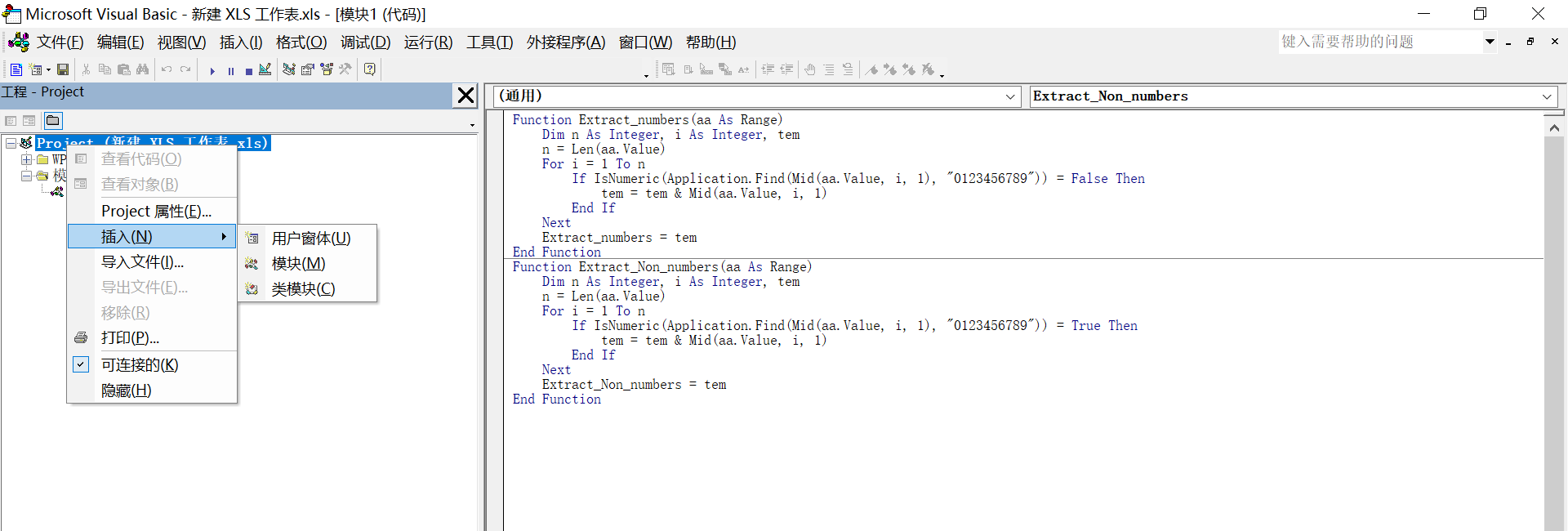2.1 提取非数字
Function Extract_numbers(aa As Range)
Dim n As Integer, i As Integer, tem
n = Len(aa.Value)
For i = 1 To n
If IsNumeric(Application.Find(Mid(aa.Value, i, 1), "0123456789")) = False Then
tem = tem & Mid(aa.Value, i, 1)
End If
Next
Extract_numbers = tem
End Function

在表格中输入对应公式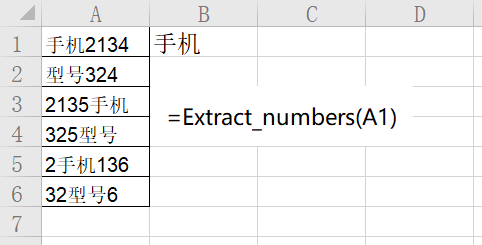2.2 提取数字
Function Extract_Non_numbers(aa As Range)
Dim n As Integer, i As Integer, tem
n = Len(aa.Value)
For i = 1 To n
If IsNumeric(Application.Find(Mid(aa.Value, i, 1), "0123456789")) = True Then
tem = tem & Mid(aa.Value, i, 1)
End If
Next
Extract_Non_numbers = tem
End Function

在表格中输入对应公式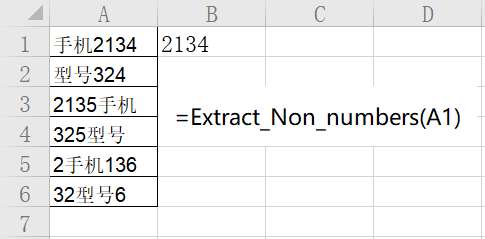3、宏程序法
按Alt+F11，打开VBA编辑器插入模块，在代码窗口中粘贴代码。 然后关闭VBA编辑器，返回Excel界面，然后按Alt+F8打开“宏”对话框，选择对应宏执行即可。
3.1 提取非数字
Sub extra_No()
Dim d As Object, arr, brr, i&
Dim sr As String
Set d = CreateObject("scripting.dictionary")
Set regex = CreateObject("VBScript.RegExp")
arr = [a1:a15]
brr = [b1:b15]
For i = 1 To UBound(brr)
sr = Range("a" & i)
With regex
.Global = True
.Pattern = "\d"
'这里也可以写成.Pattern = "[\u4e00-\u9fa5]"
Range("b" & i) = .Replace(sr, "")
End With
With [b1:b15]
.NumberFormat = "General"
End With
Next
End Sub

3.2 提取数字
Sub extra_No()
Dim d As Object, arr, brr, i&
Dim sr As String
Set d = CreateObject("scripting.dictionary")
Set regex = CreateObject("VBScript.RegExp")
arr = [a1:a15]
brr = [b1:b15]
For i = 1 To UBound(brr)
sr = Range("a" & i)
With regex
.Global = True
.Pattern = "\D"
Range("b" & i) = .Replace(sr, "")
End With
With [b1:b15]
.NumberFormat = "General"
End With
Next
End Sub

参考： 他山之石——在VBA中使用正则表达式-Part2（Regular Expression） 提取一个单元格中的文本(非数字)-自定义函数
展开全文excel
• VBA示例函数之 求人不如自已动手 提取定串中汉字，供初学者参考，大牛勿进~~~~~~~
• 实例需求：提取@之间的纯数字(无小数点)，并将结果累计求和。 测试字符串：abc100@<font color=blue>200</font>@300\$def400ghj@<font color=blue>500</font>@600正则 JAVASCRIPT
• 实例需求：只提取字符串中2位~4位的数字(无小数点)。 测试字符串：6688-部门员工共10人与2019年6月成功完成销售额889966订单数689 这次的话题有些太简单了吧！？任何学习正则的同学基本上首先学会的就是这个知识点，...正则 REGEX
• '示例:=myget(srg,1,3)'从第 3 位开始取出中文字符' =myget(srg,2)'从第 1 位开始...=myget(srg,,5)'从第 5 位开始取出数字，第 2个参数省略默认为 0' =myget(srg) '第 2、3 个参数都省略，默认为从第 1 位取出所有数字
• 提取字符中指定类型的字符_Excel VBA应用.rar,在实际工作中经常需要从字符串中提取指定类型的字符，假设目标字符串为“I Love Excel Home中123-45文 论67890坛”，利用本例介绍的代码能够提取其中的字母、数字和中文...Excel应用
• 这是我个人运用Excel VBA在EXcel中实现的提取单元格中特定文字，并用”.“来标注此单元格是否存在这个字符的小程序。
• Sub OptionalFeatureLicense() Dim Fs As Object, Ft As Object, S As String Set Fs = CreateObject("Scripting.FileSystemObject") Set d = CreateObject("scripting.dictionary") filePath = Th...
• VBA如何实现数字和文字提取并将数字处理成没有前面0的格式
• Sub tiqu() '提取文件夹下所有文件名[张志晨] '路径由浏览文件夹根据需要指定。这样灵活！ Dim strFileName　As　String'文件名
• 通过vba在excel中实现对单元格内数字、字母的暴力提取excel
• 问题：提取check之后的数字 ...Sub 提取数字() Dim regexp As New regexp With regexp .Pattern = "check\s*(\d+)" End With With Worksheets("sheet1") r = .Cells(.Rows.Count, 1).End(xlUp).Row ar...
• VBA数据处理例子 主要是提取数字，中英文的处理方法
• Function RegTest(ByVal sText As String) As String '定义正则表达式对象 Dim oRegExp As Object '定义匹配字符串集合对象 Dim oMatches As Object '创建正则表达式 Dim a As String ...正则表达式 字符串
• VBA里当读第一行时得用left(str1,6)读出来的才是姓名那个字段，读第二行时就得用left(str2,5)才正确了。问题在于汉字并不止这两个或三个字，我的文本文件里汉字数量有很大变化的，想了好多办法都不能很好解决。 ...
• 实例需求：待处理字符串如下。 >ABC1550R71PF1333K, 33nF,50V; X117R/ 0402 处理规则：仅提取其中如下图黄色部分，注意不提取第一个逗号之前的任何4位数字正则表达式 正则 正则匹配 RegExp
• 实例需求： 待处理字符串： >西装，皮带，领带各10，西装20上衣30，西装40上衣50西装皮带领带各60，西装70上衣80，西装90上衣80，70西装60上衣，...处理规则：仅提取其中包含“xx各yy”的部分，如下图黄色部分所示。正则表达式 正则
• 实例需求：数据保存在A列中，需要将其中的日期和金额分别提取至B列和C列。其中日期也两种不同格式，金额可能包含小数点、千分符和货币标识。 示例代码如下。 Sub RegExp_Date_Num() Dim Res() Dim objRegEx As ...正则 Excel Regex 日期
• 实例需求：数据序列使用减号连接，其中序列中包含重复元素。需要提取非重复元素，并使用减号连接，对于重复元素保留...这个字符提取规则相对简单，直接使用VBA方法也可以实现。 Sub VBA_DEMO_0725() Dim arrData ...正则 REGEX 重复值 唯一值
• VBA示例之 获取一个单元格中有指定字体颜色部份数据，供初学者参考，大牛勿进~~~~~~~
• 本次测试选用了三种不同的列数递增方式，区别如下： 1、提取单元格所在地址字符串，使用split()函数进行拆分后提取，简单易懂。 2、将单元格所在列数转换成字母表示。转载自...excel
• 实例需求：数据保存在A列中，需要在其中提取多组数据分别写入B：E列中。如果A列数据以反斜杠结尾，例如A4单元格100g787×1092-白牛皮沙龙纹A/，提取物料名称时需要去除末尾的反斜杠；对于非反斜杠结尾的数据，年提取...正则 Excel Regex
• 实例需求：待处理字符串如下，需要提取其中被标记颜色的部分，注意数字序号之后的符号有多种。 示例代码如下。 Sub Demo() Dim strWord As String Dim objRegExp As Object, objMH As Object Set objRegExp = ...正则表达式 正则 正则匹配
• 在各个平台上和大家分享VBA代码已经很久了，我们知道，VBA是Visual Basic for Applications的简称，是一种寄生语言，是微软开发出来在其桌面应用程序中，可以执行自动化任务的编程语言。它主要用来扩展Windows的应用......